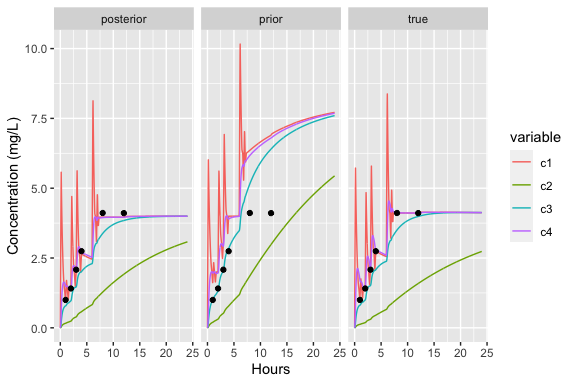# Introduction

Target-controlled infusion (TCI) systems calculate infusion rates required to reach target concentrations or effects within a patient. Where pharmacokinetic (PK) and pharmacodynamic (PD) models describe a time course of concentrations or effects, respectively, associated with a series of doses, TCI algorithms calculate the inverse relationship: what doses must be administered to achieve target responses?

The tci package implements TCI algorithms for PK and PK-PD models for drugs described by compartmental models and administered via intravenous infusion. The package provides closed-form solutions for one, two, or three compartment mammillary models (i.e., all peripheral compartments are joined to a central compartment), as well as a three-compartment model with an adjoining effect-site. PK model code is based on solutions published by Abuhelwa, Foster, and Upton (2015) and models are implemented in C++ via Rcpp. TCI algorithms for plasma- and effect-site targeting are implemented based on work by Jacobs (1990) and Shafer and Gregg (1992), respectively. Users can specify alternative PK models or TCI algorithms. See the custom vignette for further details.

library(tci)
library(ggplot2)   # ggplot for plotting
library(gridExtra) # arrangeGrob to arrange plots
library(reshape2)  # melt function

## pkmod and poppkmod object classes

The tci package is built around S3 classes pkmod and poppkmod, created with the functions pkmod and poppkmod, respectively. pkmod objects serve as containers for 1) functions implementing the structural PK model (e.g., a 1-compartment model with first-order elimination) and the PD model, if applicable, 2) the parameters for the respective functions, and 3) initial concentrations, and 4) information relevant for simulating observations or implementing TCI control, such as the compartment number associated with observations or with an effect-site. poppkmod are wrapper objects that contain one or more pkmod objects associated with published population PK models: the Marsh, Schnider, and Eleveld models for propofol, and the Minto, Kim, and Eleveld models for remifentanil.

Both pkmod and poppkmod objects have associated predict and simulate methods that can be used to predict concentrations and simulate observations (PK or PD) given an infusion schedule. Infusion schedules, in turn, are created either manually via inf_manual or by applying a TCI algorithm to reach designated targets via inf_tci. pkmod objects are additionally equipped with a update method that allows for model components (e.g., parameter values, initial concentrations) to be easily modified. Both predict and simulate methods pass additional arguments via the ellipses argument, ..., to update.pkmod to readily allow for prediction or simulation under different conditions.

Examples in this vignette will focus on illustrating the lower-level functions of tci applied to pkmod objects. See the vignette on population PK models for illustration of higher-level functions and applications to population PK models for propofol and remifentanil.

# Examples

Equations implementing 1-,2-,3-compartment and 3-compartment-effect structural PK models are included in the tci package. The function pkmod will automatically infer the correct structure based on the parameter names.

# 1-compartment model
(mod1cpt <- pkmod(pars_pk = c(cl = 10, v = 15)))
#> tci pkmod object
#> See ?update.pkmod to modify or add elements
#>
#> PK model
#>  1-compartment PK model
#>  PK parameters: cl = 10, v = 15
#>  Initial concentrations: (0)
#>  Plasma compartment: 1
#>  Effect compartment: 1
#>
#> Simulation
#>  Multiplicative error SD: 0
#>  Logged response: FALSE
# 3-compartment model with effect site
(mod3ecpt <- pkmod(pars_pk = c(cl = 10, q2 = 2, q3 =20, v = 15, v2 = 30, v3 = 50, ke0 = 1.2)))
#> tci pkmod object
#> See ?update.pkmod to modify or add elements
#>
#> PK model
#>  4-compartment PK model
#>  PK parameters: cl = 10, q2 = 2, q3 = 20, v = 15, v2 = 30, v3 = 50, ke0 = 1.2
#>  Initial concentrations: (0,0,0,0)
#>  Plasma compartment: 1
#>  Effect compartment: 4
#>
#> Simulation
#>  Multiplicative error SD: 0
#>  Logged response: FALSE

Acceptable parameter names can be viewed by calling list_parnms(). Less-commonly used parameters, such as clearance from a peripheral compartment, are also permissible.

# acceptable parameter names
list_parnms()
#> Acceptable names for 'pars_pk' vector (case-insensitive)
#>
#> First compartment options
#>  Central volume: 'v','v1'
#>  Elimination: 'cl','cl1','k10','ke'
#>
#> Second compartment options
#>  Peripheral volume: 'v2'
#>  Transfer: 'q','q2','k12','k21'
#>  Elimination: 'cl2','k20'
#>
#> Third compartment options
#>  Second peripheral volume: 'v3'
#>  Transfer: 'q3','k13','k31'
#>  Elimination: 'cl3','k30'
#>
#> Effect-site
#>  Elimination: 'ke0'

Elements of pkmod objects can be modified through an update.pkmod method. Perhaps most usefully, this allows for partial modifications to PK-PD parameters. For example, the effect-site equilibrium constant can be easily updated.

update(mod3ecpt, pars_pk = c(ke0 = 0.9), init = c(1,0.2,0.3,1))
#> tci pkmod object
#> See ?update.pkmod to modify or add elements
#>
#> PK model
#>  4-compartment PK model
#>  PK parameters: cl = 10, q2 = 2, q3 = 20, v = 15, v2 = 30, v3 = 50, ke0 = 0.9
#>  Initial concentrations: (1,0.2,0.3,1)
#>  Plasma compartment: 1
#>  Effect compartment: 4
#>
#> Simulation
#>  Multiplicative error SD: 0
#>  Logged response: FALSE

Most functions in the tci package pass additional arguments to update.pkmod allowing for easy modification of pkmod objects as needed.

## Infusion schedules

An infusion schedule is required to for predict and simulate methods. This schedule should be a matrix with column labels “begin”, “end”, and “infrt”, indicating infusion begin times, end times, and infusion rates. It can be created directly by the user, or outputted by the inf_manual or inf_tci functions. In the former function, the user specifies infusion start times, durations, and infusion rates.

# single infusion
(single_inf <- inf_manual(inf_tms = 0, duration = 0.5, inf_rate = 100))
#>      begin end inf_rate
#> [1,]     0 0.5      100
# multiple infusions
(multi_inf <- inf_manual(inf_tms = c(0,3,6), duration = c(1,0.5,0.25), inf_rate = 100))
#>      begin  end inf_rate
#> [1,]   0.0 1.00      100
#> [2,]   1.0 3.00        0
#> [3,]   3.0 3.50      100
#> [4,]   3.5 6.00        0
#> [5,]   6.0 6.25      100

Typically, however, the inf_tci will be used to calculate infusion rates required to reach specified targets. inf_tci requires 1) a set of target concentrations (or PD response values) and corresponding times at which the target is set, and 2) a pkmod object. It has “plasma” and “effect” settings, implementing the Jacobs and Shafer algorithms, respectively. Custom algorithms can be specified through the custom_alg argument. See the vignette on custom models and algorithms for more details.

# plasma targeting for one-compartment model
inf_1cpt <- inf_tci(target_vals = c(2,3,4,4), target_tms = c(0,2,3,10),
pkmod = mod1cpt, type = "plasma")
#>        begin     end inf_rate Ct c1_start c1_end
#> [1,] 0.00000 0.16667 190.1851  2        0      2
#> [2,] 0.16667 0.33333  20.0000  2        2      2
#> [3,] 0.33333 0.50000  20.0000  2        2      2
#> [4,] 0.50000 0.66667  20.0000  2        2      2
#> [5,] 0.66667 0.83333  20.0000  2        2      2
#> [6,] 0.83333 1.00000  20.0000  2        2      2

# effect-site targeting for three-compartment effect site model
inf_3ecpt <- inf_tci(target_vals = c(2,3,4,4), target_tms = c(0,2,3,10),
pkmod = mod3ecpt, type = "effect")
#>        begin     end inf_rate Ct c1_start   c2_start  c3_start  c4_start
#> [1,] 0.00000 0.16667 643.6921  2 0.000000 0.00000000 0.0000000 0.0000000
#> [2,] 0.16667 0.33333   0.0000  2 6.033672 0.03532348 0.2079682 0.5966263
#> [3,] 0.33333 0.50000   0.0000  2 4.301795 0.09138590 0.5235366 1.4096671
#> [4,] 0.50000 0.66667   0.0000  2 3.136188 0.13103476 0.7267367 1.8178340
#> [5,] 0.66667 0.83333  19.3835  2 2.350071 0.15960595 0.8548449 1.9785480
#> [6,] 0.83333 1.00000   0.0000  2 2.000000 0.18174014 0.9390928 2.0109479
#>        c1_end     c2_end    c3_end    c4_end
#> [1,] 6.033672 0.03532348 0.2079682 0.5966263
#> [2,] 4.301795 0.09138590 0.5235366 1.4096671
#> [3,] 3.136188 0.13103476 0.7267367 1.8178340
#> [4,] 2.350071 0.15960595 0.8548449 1.9785480
#> [5,] 2.000000 0.18174014 0.9390928 2.0109479
#> [6,] 1.586605 0.19939667 0.9931813 1.9678507

By default, plasma- and effect-targeting algorithms are updated in increments of 1/6. If a PK model elimination parameters have units of minutes (as do commonly used models for the anesthetic propofol), this will correspond to updating TCI targets at 10-second intervals. If elimination rates are in different units, such as hours, then the TCI update frequency should be modified by the argument dtm.

## Predict and simulate methods

The infusion schedule can be applied to the pkmod object using predict.pkmod or simulate.pkmod methods to predict concentrations or simulate observations, respectively. Using the three-compartment model as illustration

# prediction/observation times
tms_pred <- seq(0,10,0.01)
tms_obs <- c(0.5,1,2,4,6,10)

pre <- predict(mod3ecpt, inf = inf_3ecpt, tms = tms_pred)
obs <- simulate(mod3ecpt, seed = 1, inf = inf_3ecpt, tms = tms_obs, sigma_mult = 0.2)

# plot results
dat <- data.frame(time = tms_pred, plasma (3 cmpt) = pre[,"c1"],
effect (ke0=1.2) = pre[,"c4"],
check.names = FALSE)
datm <- melt(dat, id = "time")
dat_obs <- data.frame(time = tms_obs, con = obs, variable = "plasma (3 cmpt)")

p <- ggplot(datm, aes(x = time, y = value, color = variable)) +
geom_line() +
geom_point(data = dat_obs, aes(x = time, y = con)) +
xlab("Minutes") + ylab("Concentration (mg/L)")
p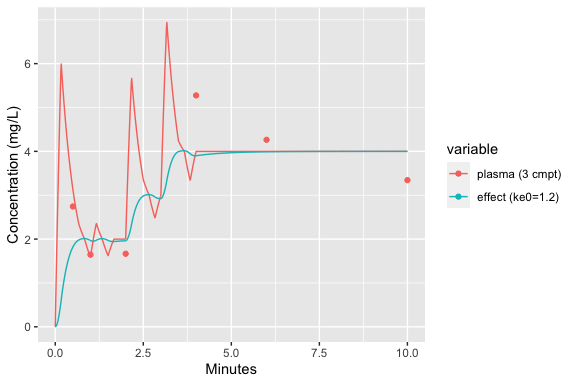Notably, the pkmod object used in the predict and simulate methods does not need to be the same as the one used to calculate the infusion schedule. This permits the user to evaluate the effect of model misspecification either 1) by passing different parameter values to update.pkmod via predict.pkmod or simulate.pkmod, or 2) by using a different pkmod object.

To illustrate the parameter misspecification, we can evaluate predictions with a new effect-site equilibrium constant.

# evaluate with different ke0 parameter
pre_misspec <- predict(mod3ecpt, inf = inf_3ecpt, tms = tms_pred,
pars_pk = c(ke0 = 0.8))
dat_misspec <- data.frame(pre_misspec, variable = "effect (ke0=0.8)", time = tms_pred)
p + geom_line(data = dat_misspec, aes(x = time, y = c4, color = variable))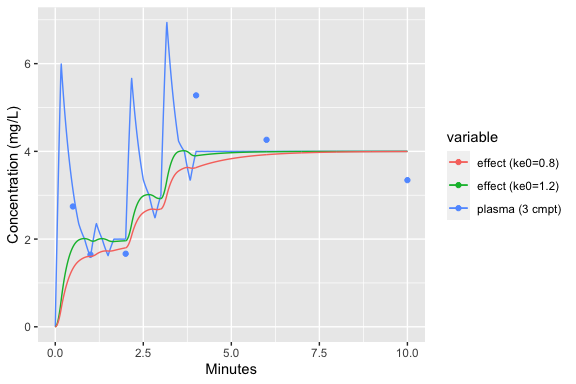To illustrate structural model misspecification, we can consider the case where PK are described by a one-compartment model, but infusions were calculated according to a three-compartment model.

# predicted concentrations
pre_1cpt <- predict(mod1cpt, inf = inf_3ecpt, tms = tms_pred)
dat_1cpt <- data.frame(pre_1cpt, variable = "plasma (1 cmpt)", time = tms_pred)
# simulated observations
obs_1cpt <- simulate(mod1cpt, seed = 1, inf = inf_3ecpt, tms = tms_obs, sigma_mult = 0.2)

p + geom_line(data = dat_1cpt, aes(x = time, y = c1, color = variable)) +
geom_point(data = data.frame(time = tms_obs, con = obs_1cpt, variable = "plasma (1 cmpt)"),
aes(x = time, y = con), inherit.aes = FALSE, color = "green4")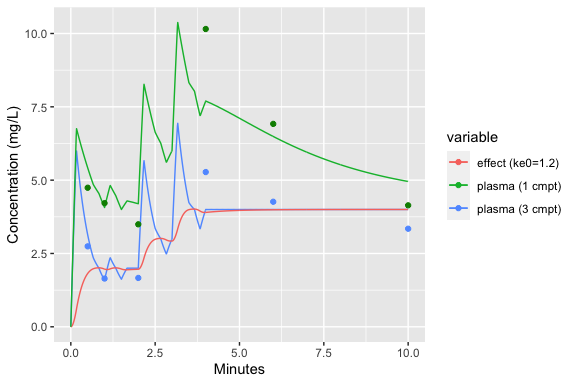## Extensions to PK-PD models

All of the functions in tci can be extended to include pharmacodynamic (PD) models. Unlike PK models, the equations describing PD models are typically invertible, allowing one to readily calculate the target effect-site concentration associated with a desired effect. The user, therefore, supplies to a pkmod functions implementing the PD response (i.e., compute response from concentrations), and its inverse (i.e., concentrations from a response), as well as associated parameter values.

Four-parameter E-max models are commonly used to describe PD responses and are implemented in tci. E-max models describe a response in terms of its minimum and maximum values, emx and e0, respectively, the concentration associated with 50% effect, c50, and the slope of the dose-response curve at c50, gamma. In anesthesia, the Bispectral Index (BIS) is a commonly used measurement of a patient’s depth of hypnosis and is often described by an E-max model. BIS is derived from EEG measurements and calibrated to vary between BIS=100, indicating a fully-alert state, and BIS=0, in which little brain activity is registered. BIS values between 40 and 60 typically indicate that a patient is sufficiently sedated for general anesthesia.

modpd <- update(mod3ecpt, pdfn = emax, pdinv = emax_inv,
pars_pd = c(e0 = 100, emx = 100, c50 = 3.5, gamma = 2.2))

PD targets are passed along with the updated pkmod to inf_tci, which will assume values are PD values (unless overridden by the ignore_pd argument of inf_tci).

inf_pd <- inf_tci(target_vals = c(70,60,50,50), target_tms = c(0,2,3,10), pkmod = modpd, type = "effect")

We can then similarly use predict.pkmod and simulate.pkmod methods to predict and simulate PD responses. BIS measurements may be collected at a rate of one observation per 10-20 seconds, depending on the BIS device settings.

# predict responses
pre_pd <- predict(modpd, inf = inf_pd, tms = tms_pred)
# pd observations: 10 sec = 1/6 min
tms_pd_obs <- seq(1/6,10,1/6)
# simulate responses with additive error and parameter misspecification
obs_pd <- simulate(modpd, seed = 1, inf = inf_pd, tms = tms_pd_obs, sigma_add = 5,
pars_pk = c(ke0 = 0.7), pars_pd = c(c50 = 3, gamma = 1.8))

# plot results
dat_pd <- data.frame(time = tms_pred, plasma (3 cmpt) = pre_pd[,"c1"],
effect (ke0=1.2) = pre_pd[,"c4"],
BIS = pre_pd[,"pdresp"],
check.names = FALSE)
dat_pdm <- melt(dat_pd, id = "time")
dat_pdm$type <- as.factor(ifelse(dat_pdm$variable == "BIS", "PD","PK"))
dat_pd_obs <- data.frame(time = tms_pd_obs, BIS = obs_pd,
type = factor("PD"), variable = "BIS")
levels(dat_pdm$type) <- levels(dat_pd_obs$type) <- c("Bispectral Index", "Concentration (mg/L)")

ggplot(dat_pdm, aes(x = time, y = value, color = variable)) +
facet_wrap(type~., scales = "free", nrow = 2) +
geom_line() +
geom_point(data = dat_pd_obs, aes(x = time, y = BIS)) +
xlab("Minutes") + ylab("")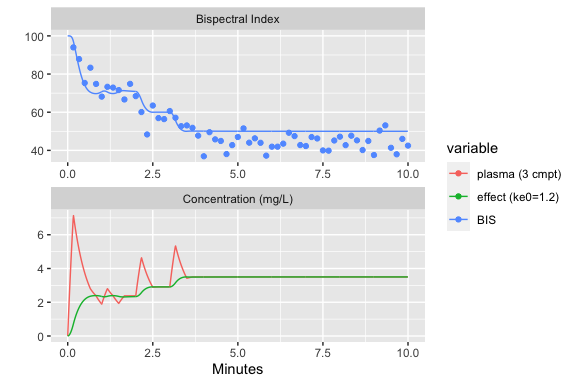## Open- and closed-loop control

Simulations with potential model misspecification are most easily implemented using the function simulate_tci which can be used for both pkmod and poppkmod classes. Required arguments to simulate_tci are 1) a prior PK model (pkmod_prior) that is used to calculate infusion rates and may be updated throughout the simulation if update times are provided, 2) a true PK model (pkmod_true) that is used to simulate observations, 3) TCI target values, 4) TCI target times, and 5) times to simulate observations. If update times are specified then Bayesian updates will be performed to update parameters based on the (simulated) data available at each time. Data processing delays can be incorporated through the argument delay.

To illustrate open-loop control, we simulate PK responses from a three-compartment model at times 1, 2, 3, 4, 8, and 12 over a 24 hour period in which effect-site targeting is used and the target concentration is raised from 2 mg/L to 4 mg/L.

mod_true  <- update(mod3ecpt, pars_pk = c(cl = 20, q2 = 1.5, ke0 = 1.8))
sim_ol <- simulate_tci(pkmod_prior = mod3ecpt,
pkmod_true = mod_true,
target_vals = c(2,3,4,4),
target_tms = c(0,2,3,24),
obs_tms = c(1,2,3,4,8,12),
seed = 1)
ggplot(melt(sim_ol$resp, id.vars = c("time","type"))) + geom_line(aes(x = time, y = value, color = variable)) + geom_point(data = sim_ol$obs, aes(x = time, y = obs)) +
facet_wrap(~type) +
labs(x = "Hours", y = "Concentration (mg/L)")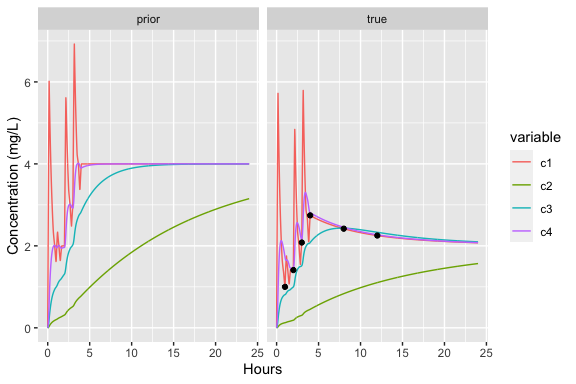Closed-loop control is implemented by specifying a set of update times. For model parameters to be updated, pkmod_prior must have an “Omega” matrix specifying the variability in each parameter. This matrix is used as the prior variance-covariance matrix in the updates, while the prior model parameters are used as the prior point estimates.

Using the example above, we simulate samples drawn at 1, 2, 4, and 8 hours, with a processing time of 4 hours for each sample.

mod3ecpt <- update(mod3ecpt, sigma_mult = 0.2,
Omega = matrix(diag(c(1.2,0.6,1.5,0.05)), 4,4,
dimnames = list(NULL, c("cl","q2","v","ke0"))))
sim_cl <- simulate_tci(pkmod_prior = mod3ecpt,
pkmod_true = mod_true,
target_vals = c(2,3,4,4),
target_tms = c(0,2,3,24),
obs_tms = c(1,2,3,4,8,12),
update_tms = c(6,12,16),
delay = 0,
seed = 1)
ggplot(melt(sim_cl$resp, id.vars = c("time","type"))) + geom_line(aes(x = time, y = value, color = variable)) + geom_point(data = sim_cl$obs, aes(x = time, y = obs)) +
facet_wrap(~type) +
labs(x = "Hours", y = "Concentration (mg/L)")